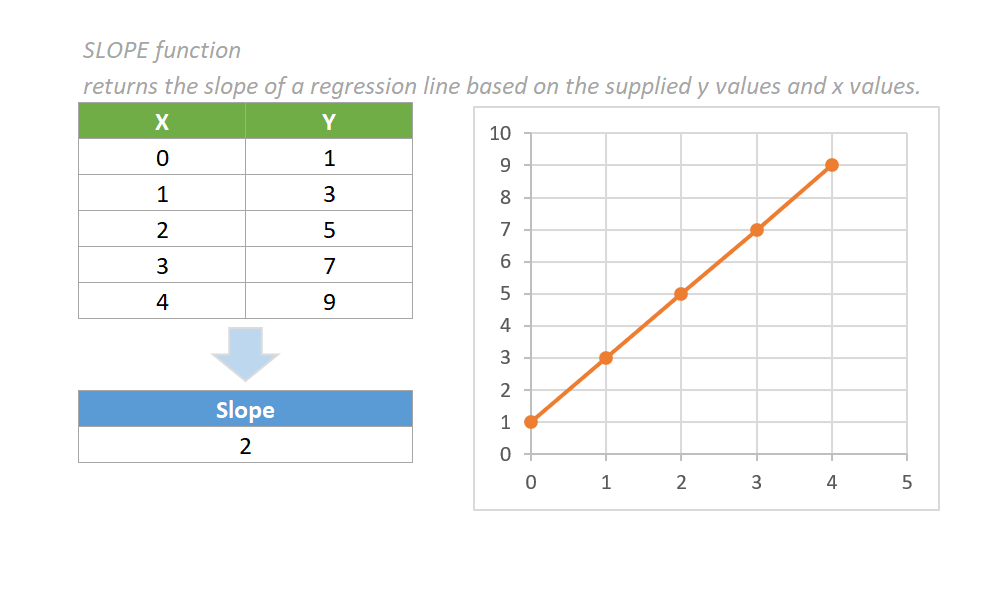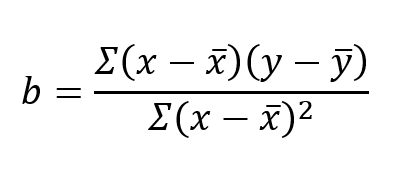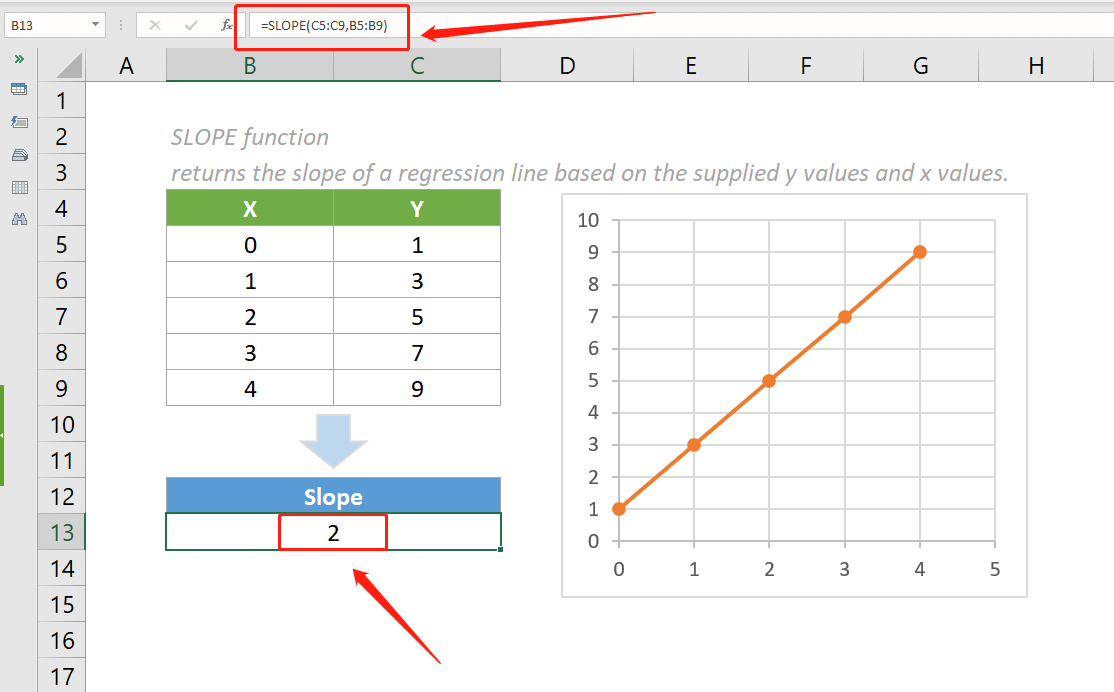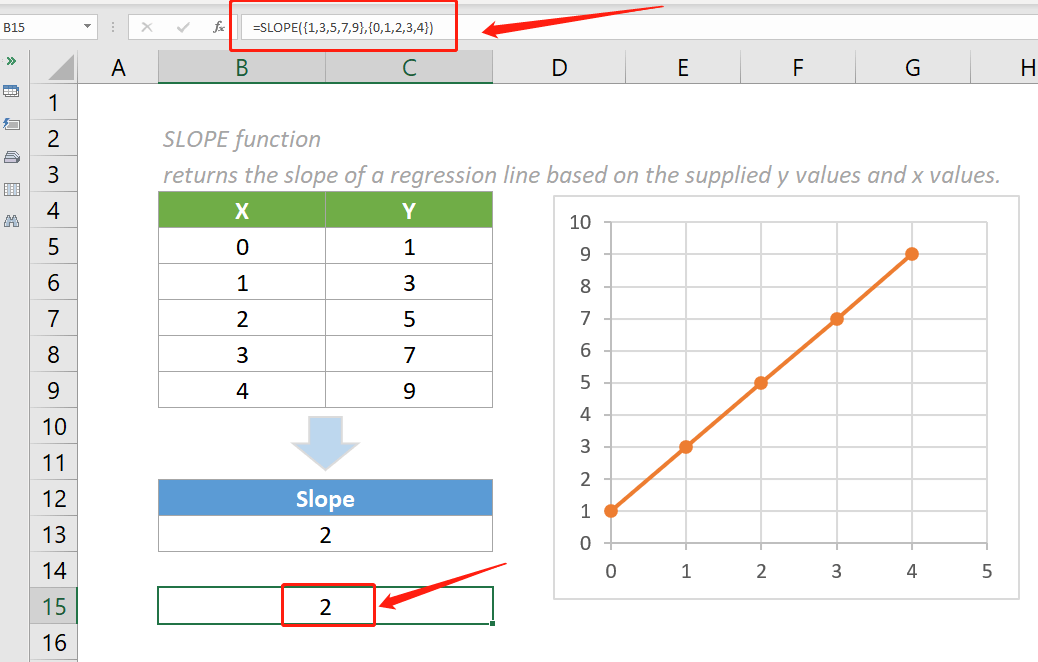## Excel SLOPE Function

The SLOPE function returns the slope of a regression line based on the supplied y values and x values. The slope is the ratio of rise and run, which means the vertical distance divided by the horizontal distance between any two points on the line.#### Syntax

=SLOPE (known_y's, known_x's)

#### Arguments

• Known_y's (required): An array or range of numeric y values.
• Known_x's (required): An array or range of numeric x values.

#### Return value

The SLOPE function returns a numeric value.

#### Function notes

1. Arguments in the SLOPE function can be supplied as range names, arrays or ranges that contain numbers.
2. SLOPE function ignores empty cells or cells that contain text or logical values.
3. Cells with zero values will be included in the calculation.
4. The #DIV/0! error value occurs when either of the following conditions is met:
• only one y value and one x value are supplied.
• the supplied know_y’s or know_x’s arrays is empty.
5. The #N/A error value occurs if the numbers of the supplied y values and x values are different.
6. The equation of the SLOPE function is:#### Examples

To calculate the slope of a regression line based on the supplied y values and x values provided in the below table, please copy the formula below into cell B13, then press the Enter key to get the result.

=SLOPE(C5:C9, B5:B9)Notes:

1. The arguments in the above formula are supplied as two ranges that contain multiple values.
2. The arguments can also be supplied as constant arrays. An array constant is a hard-coded set of values in an Excel formula, appearing in curly braces {}. The above formula can be changed to:

=SLOPE({1,3,5,7,9}, {0,1,2,3,4})#### Relative Functions:

• Excel EVEN Function
The EVEN function rounds numbers away from zero to the nearest even integer.

• Excel EXP Function
The EXP function returns the result of the constant e raised to the nth power.

### The Best Office Productivity Tools

#### Kutools for Excel - Helps You To Stand Out From Crowd

 Popular Features: Find, Highlight or Identify Duplicates  |  Delete Blank Rows  |  Combine Columns or Cells without Losing Data  |  Round without Formula ... Super VLookup: Multiple Criteria  |  Multiple Value  |  Across Multi-Sheets  |  Fuzzy Lookup... Adv. Drop-down List: Easy Drop Down List  |  Dependent Drop Down List  |  Multi-select Drop Down List... Column Manager: Add a Specific Number of Columns  |  Move Columns  |  Toggle Visibility Status of Hidden Columns  |  Compare Columns to Select Same & Different Cells ... Featured Features: Grid Focus  |  Design View  |  Big Formula Bar  |  Workbook & Sheet Manager | Resource Library (Auto Text)  |  Date Picker  |  Combine Worksheets  |  Encrypt/Decrypt Cells  |  Send Emails by List  |  Super Filter  |  Special Filter (filter bold/italic/strikethrough...) ... Top 15 Toolsets:  12 Text Tools (Add Text, Remove Characters ...)  |  50+ Chart Types (Gantt Chart ...)  |  40+ Practical Formulas (Calculate age based on birthday ...)  |  19 Insertion Tools (Insert QR Code, Insert Picture from Path ...)  |  12 Conversion Tools (Numbers to Words, Currency Conversion ...)  |  7 Merge & Split Tools (Advanced Combine Rows, Split Excel Cells ...)  |  ... and more

Kutools for Excel Boasts Over 300 Features, Ensuring That What You Need is Just A Click Away...#### Office Tab - Enable Tabbed Reading and Editing in Microsoft Office (include Excel)

• One second to switch between dozens of open documents!
• Reduce hundreds of mouse clicks for you every day, say goodbye to mouse hand.
• Increases your productivity by 50% when viewing and editing multiple documents.
• Brings Efficient Tabs to Office (include Excel), Just Like Chrome, Edge and Firefox.